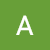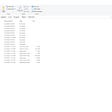# Transforming a pre-formatted excel report into a tabular format without macros — Reporting system part 2

`# Import librariesimport pandas as pdimport datetimefrom os import listdirfrom os.path import isfile, joinimport reimport timefrom airflow.models import VariableRAW_DATA_LOCATION = Variable.get('raw_data_location')STAGING_DATA_LOCATION = Variable.get('staging_data_location')# Set Pandas to display 500 rowspd.set_3('display.max_rows', 500)# Set Folder to usefolder = RAW_DATA_LOCATIONdef cleanfiles(folder):    #List files in folder    folderfiles = [f for f in listdir(folder) if isfile(join(folder,    f))]    files = []    #Remove hidden files    for file in folderfiles:        if not file.startswith('~\$'):            files.append(file)    #Return only excel files    files = [file for file in files if file.endswith('xlsx')]    return files`
`def cleandata(folder):    df_list = []  for file in cleanfiles(folder):   #Read in excel files        df = pd.read_excel(folder+'/'+file, skiprows=9)        #Drop empty column        df = df.drop('Unnamed: 0',axis=1)        # Drop all empty rows        df = df.dropna(how='all',axis=0)        # Fill NAN cells with 0        df['18'].fillna(0, inplace=True)        df['19'].fillna(0, inplace=True)        # Drop any remaining rows with NAN values in any cell        df = df.dropna(how='any', axis=0)        # Drop duplicate rows ( This eliminates the duplicate headers from the separate files)        df = df.drop_duplicates(keep=False)        #Add date in new column        date_time_str = file.split('.')        filedate = datetime.datetime.strptime(date_time_str, '%Y-%m-%d')        df['date'] = filedate        # Rename and change types        df['2'] = df['2'].astype('int64')        df['3'] = df['3'].astype('int64')        df['4'] = df['4'].astype('int64')        df['7'] = df['7'].astype('float')        df['8'] = df['8'].astype('float')        df['9'] = df['9'].astype('int64')        df['10'] = df['10'].astype('float')        df['11'] = df['11'].astype('int64')        df['12'] = df['12'].astype('int64')        df['13'] = df['13'].astype('int64')        df['14'] = df['14'].astype('int64')        df['15'] = df['15'].astype('int64')        df['16'] = df['16'].astype('int64')        df['17'] = df['17'].astype('int64')        df['18'] = df['18'].astype('int64')        df['19'] = df['19'].astype('int64')        df.rename(columns =          {'12':'9-1'          ,'13':'9-2'          ,'14':'9-3'          },inplace=True)        #Append to list of data frames        df_list.append(df) #Concatenate newly clean dataframes into one large dataframe df = pd.concat(df_list)    return df`
`def category_df(folder):    category_df_list = []    def hasNumbers(inputString):                return bool(re.search(r'\d', inputString))    def isDept(x):    # 1 refers to column 1 which is the sub-category       if x =='1':          return True`
`def category_df(folder):    category_df_list = []def hasNumbers(inputString):                return bool(re.search(r'\d', inputString))def isDept(x):        if x =='1':            return Truefor file in cleanfiles(folder):        #Read in excel files        categorydf = pd.read_excel(folder+'/'+file, skiprows=6)        categorydf = categorydf['Unnamed: 1'].reset_index()        categorydf = categorydf.fillna(method='ffill')        categorydf.drop('index',axis=1, inplace=True)        #Add column to determine if column has integer in string        categorydf['bool'] = categorydf['Unnamed: 1'].map(lambda x:           hasNumbers(x))        #If the column contains an integer use this value as the column value for the category column         categorydf['Category'] = categorydf.apply(lambda x: x['Unnamed: 1'] if x['bool'] ==True else None, axis=1 )#forward fill categories to associate the sub category with the category         categorydf.fillna(method='ffill', inplace=True)#Create boolean column to decide if the column is the column header        categorydf['isDept?'] = categorydf['Unnamed: 1'].map(lambda x: isDept(x))        categorydf.drop_duplicates(inplace=True)#Filter out any rows with the sub-category header         mask = (categorydf['bool'] ==False) & (categorydf['isDept?'] != True)        categorydf = categorydf[mask]        categorydf = categorydf.copy()        categorydf.rename(columns={'Unnamed: 1': '1'}, inplace=True)        categorydf['Category'] = categorydf['Category'].map(lambda x: re.sub(r'\d+', '', x))        categorydf['Category'] = categorydf['Category'].map(lambda x: x[1:])        categorydf.drop(['bool','isDept?'], axis=1,inplace=True)        category_df_list.append(categorydf)category_df = pd.concat(category_df_list)    category_df.drop_duplicates(keep='first',inplace=True)#Final result with department names and categories side by side as a look up table    category_df = category_df[['Category','1']].sort_values(by='Category', ascending=True).reset_index().drop('index',axis=1)    return category_dfdf = cleandata(folder)df.to_csv(STAGING_DATA_LOCATION+'sales_stage.csv')categorydf = category_df(folder)categorydf.to_csv(STAGING_DATA_LOCATION+'category_stage.csv')`
`# Import librariesimport pandas as pdimport datetimefrom os import listdirfrom os.path import isfile, joinfrom airflow.models import Variabledef dim_status(df):    current_status = df['6'].drop_duplicates().reset_index().drop('index',axis=1)    current_status['Status_SK'] =  range(1, len(current_status.index)+1)    current_status = current_status[['Status_SK','6']]    return current_statusdef to_integer(dt_time):    return 10000*dt_time.year + 100*dt_time.month + dt_time.daydef create_date_table(df):    start = df['date'].min()    end = df['date'].max()    df = pd.DataFrame({"Date": pd.date_range(start, end)})    df['Date_SK'] = df['Date'].map(lambda x: to_integer(x))    df["Day"] = df.Date.dt.day_name    df["Week"] = df.Date.dt.weekofyear    df["Quarter"] = df.Date.dt.quarter    df["Year"] = df.Date.dt.year    df["Year_half"] = (df.Quarter + 1) // 2    date_table = df    cols = ['Date_SK','Date','Day','Week','Quarter','Year','Year_half']    date_table = date_table[cols]    return date_tabledef dim_item(df):    mask = ['5','1','4','2','3']    df2 = df[mask].drop_duplicates().sort_values(by='5')    df2['Item_SK'] = range(1, len(df2.index)+1)    Item = df2[['Item_SK','5','1','4','2','3']]    Item = Item.merge(category_df,left_on='1', right_on='1', how='left')    Item_codes = Item['3']Item_urls = []    for code in Item_codes:        URL = 'https://www.company.com/uk/p/{}'.format(code)        Item_urls.append(URL)Image_urls = []    for code in Item_codes:        URL = 'https://media2.companyassets.com/i/company/{}/'.format(code)        Image_urls.append(URL)Item['ItemURL'] = Item_urls    Item['ImageURL'] = Image_urlsreturn Itemdef sales(df):    df = df.merge(Status, left_on='6',right_on='6', how='left').drop('6', axis=1)    cols_to_use = Item.columns.difference(df.columns)    Item[cols_to_use]    columns = ['2','3','5','4','1']    sales = df.merge(Item, left_on=columns, right_on=columns, how='left').drop(columns,axis=1)    sales.rename(columns={'date':'Date'}, inplace=True)    sales['Date']= pd.to_datetime(sales['Date'])    Date_table = Date[['Date_SK','Date']]    sales = sales.merge(Date_table,left_on='Date',right_on='Date')    cols = ['Item_SK','Status_SK','Date_SK','7', '8', '9',           '10', '11', '9-1', '9-2',           '9-3', '',           '16', '17', '18',           '19' ]    sales = sales[cols]return salesSTAGING_DATA_LOCATION = Variable.get('staging_data_location')STAR_SCHEMA_LOCATION = Variable.get('star_schema_location')staging_df = pd.read_csv(STAGING_DATA_LOCATION+'sales_stage.csv')category_df = pd.read_csv(STAGING_DATA_LOCATION+'category_stage.csv')Status = dim_status(staging_df)Item = dim_item(staging_df)Date = create_date_table(staging_df)Sales = sales(staging_df)table_dict = {'Status':Status,'Item':Item,'Date':Date,'Sales':Sales}for name,table in table_dict.items():    table.to_csv(STAR_SCHEMA_LOCATION+name+'.csv')`

## More from Alexander

Love podcasts or audiobooks? Learn on the go with our new app.

## 6 Tips for a Successful Developer Career## Dell Hiring Freshers For Software Test Engineer Position | Apply Now## HackTheBox-Armageddon## Mutable, Immutable… Everything is an Object!## Creating a New User, an S3 Bucket and granting the user access to the S3.## Enhance your SEO with Drupal Semantic Web## More from Medium## Meet The Team: Nick Wong## Innovation of Data Integration Technology in the Intelligent Era## How to Use Python & SQL to Append New Rows to a BigQuery Table Without Overwriting Your Data